# Learn to Code SQL Example – SQL Server Mathematical functions (SQRT, PI, SQUARE, ROUND, CEILING & FLOOR)# SQL Server Mathematical functions (SQRT, PI, SQUARE, ROUND, CEILING & FLOOR)

Mathematical functions are present in SQL server which can be used to perform mathematical calculations. Some commonly used mathematical functions are given below:
1. SQRT():
SQRT() function is the most commonly used function. It takes any numeric values and returns the square root value of that number.

Syntax:

`SELECT SQRT(..value..)`

Example: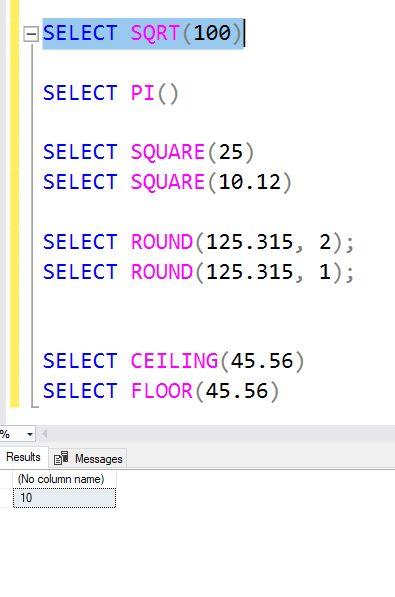2. PI(): There are calculations which require use of pi. Using pi() function, value of PI can be used anywhere in the query.

Syntax:

`SELECT PI()`

Example: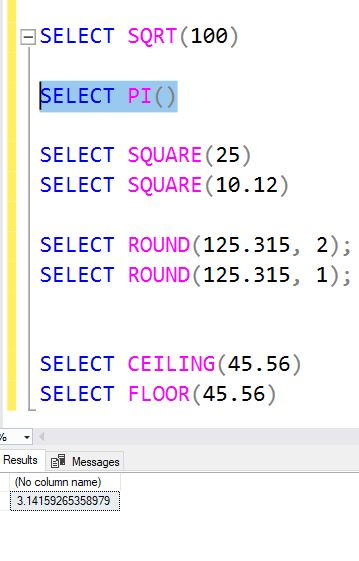3. SQUARE(): SQUARE() function is used to find the square of any number.

Syntax:

`SELECT SQUARE(..value..)`

Example: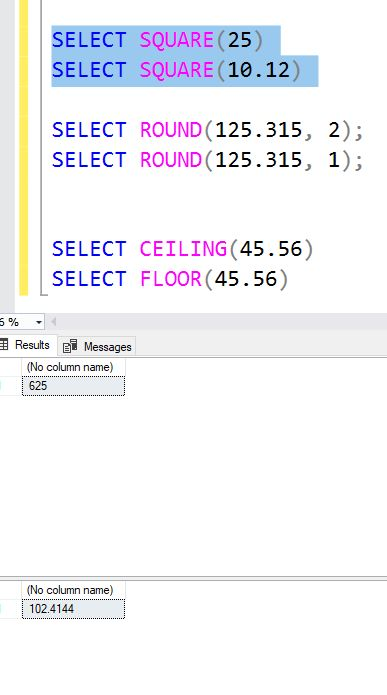4. ROUND(): ROUND() function is used to round a value to the nearest specified decimal place.

Syntax:

`SELECT ROUND(..value.., number_of_decimal_places)`

Example: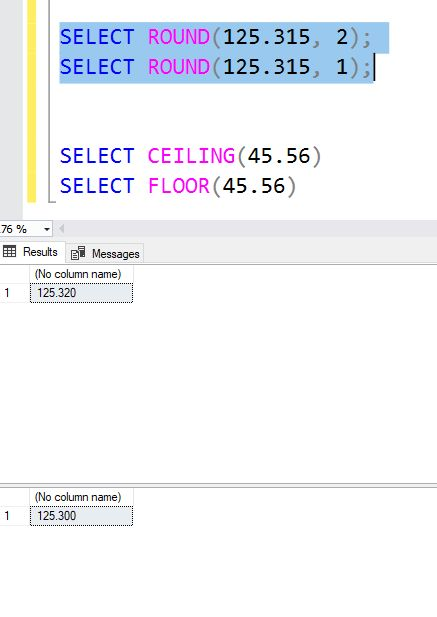5. CEILING() and FLOOR()
CEILING(): CEILING() function is used to find the next highest value (integer).

Syntax:

`SELECT CEILING(..value..)`

FLOOR(): FLOOR() function returns the next lowest value (integer).
Syntax:

`SELECT FLOOR(..value..)`

Example: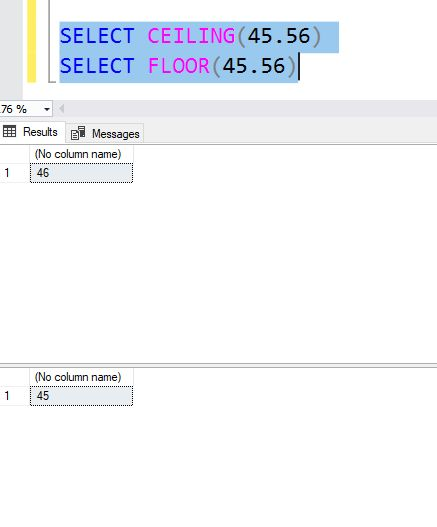# Personal Career & Learning Guide for Data Analyst, Data Engineer and Data Scientist

## Applied Machine Learning & Data Science Projects and Coding Recipes for Beginners

A list of FREE programming examples together with eTutorials & eBooks @ SETScholars

# Projects and Coding Recipes, eTutorials and eBooks: The best All-in-One resources for Data Analyst, Data Scientist, Machine Learning Engineer and Software Developer

Topics included: Classification, Clustering, Regression, Forecasting, Algorithms, Data Structures, Data Analytics & Data Science, Deep Learning, Machine Learning, Programming Languages and Software Tools & Packages.
(Discount is valid for limited time only)`Disclaimer: The information and code presented within this recipe/tutorial is only for educational and coaching purposes for beginners and developers. Anyone can practice and apply the recipe/tutorial presented here, but the reader is taking full responsibility for his/her actions. The author (content curator) of this recipe (code / program) has made every effort to ensure the accuracy of the information was correct at time of publication. The author (content curator) does not assume and hereby disclaims any liability to any party for any loss, damage, or disruption caused by errors or omissions, whether such errors or omissions result from accident, negligence, or any other cause. The information presented here could also be found in public knowledge domains.`

# Learn by Coding: v-Tutorials on Applied Machine Learning and Data Science for Beginners

Please do not waste your valuable time by watching videos, rather use end-to-end (Python and R) recipes from Professional Data Scientists to practice coding, and land the most demandable jobs in the fields of Predictive analytics & AI (Machine Learning and Data Science).

The objective is to guide the developers & analysts to “Learn how to Code” for Applied AI using end-to-end coding solutions, and unlock the world of opportunities!